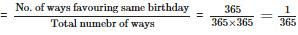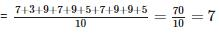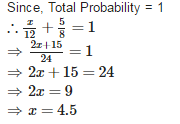Courses

# RD Sharma Test: Probability

## 25 Questions MCQ Test Mathematics (Maths) Class 10 | RD Sharma Test: Probability

Description
This mock test of RD Sharma Test: Probability for Class 10 helps you for every Class 10 entrance exam. This contains 25 Multiple Choice Questions for Class 10 RD Sharma Test: Probability (mcq) to study with solutions a complete question bank. The solved questions answers in this RD Sharma Test: Probability quiz give you a good mix of easy questions and tough questions. Class 10 students definitely take this RD Sharma Test: Probability exercise for a better result in the exam. You can find other RD Sharma Test: Probability extra questions, long questions & short questions for Class 10 on EduRev as well by searching above.
QUESTION: 1

### An unbiased die is thrown once. The probability of getting a prime number is

Solution:

Number of possible outcomes = {2, 3, 5} = 3
Number of Total outcomes = 6
∴ Probability of getting a prime number = 3/6 = 1/2

QUESTION: 2

### The probability that a non leap year will have 53 Fridays and 53 Saturdays is

Solution:

Non-leap year contains 366 days = 52 weeks + 1 day
52 weeks contain 52 Fridays and 52 weeks contain 52 Saturdays
We will get 53 Fridays or 53 Saturdays if remaining one day is a Friday and Saturday
Therefore, Total possibility is zero.
Number of Total possible outcomes = 7
Number of possible outcomes Friday and Saturday = 0
Required Probability = 0/7=0

QUESTION: 3

### A number is selected at random from 1 to 75. The probability that it is a perfect square is

Solution:

Number of possible outcomes = {1, 4, 9, 16, 25, 36, 49, 64} = 8
Number of Total outcomes = 75
∴ Probability of getting a perfect square = 8/75

QUESTION: 4

Ram and Shyam are friends. The probability that both will have the same birth day is

Solution:

Ignoring a leap year, both Ram and Shyam can have any day of the year as their birthday.
∴ Total number of possible ways in which their birthday can fall =
Also they can share the same birthday falls on same date = 365
∴ Required ProbabilityQUESTION: 5

Which of the following can not be the probability of an event?

Solution:

17/16 cannot be the probability of an event because probability of an event cannot be greater than 1.

QUESTION: 6

A die is thrown once. The probability of getting an even number and a multiple of 3 is

Solution:

Number of possible outcomes = {6} = 1
Number of Total outcomes = 6
∴ Required Probability = 1/6

QUESTION: 7

The probability of selecting a queen of diamonds when a card is drawn from well shuffled pack of 52 cards is

Solution:

Number of possible outcomes (queen of diamonds) = 1
Number of Total outcomes = 52
Required Probability = 1/52

QUESTION: 8

A piggy bank contains 100 fifty paise coins, 50 one rupee coins, 20 two rupee coins and 10 five rupee coins. One coin is drawn at random. The probability that the coin drawn will not be a five rupee coin is

Solution:

Number of total coins = 100 + 50 + 20 + 10 = 180
Number of coins except five rupee coins = 180 – 10 = 170
∴ Required Probability = 170/180 = 17/18

QUESTION: 9

A letter of English alphabets is chosen at random. The probability that the letter is consonant

Solution:

Number of consonants = 21
Number of total outcomes = 26
∴ Required Probability = 21/26

QUESTION: 10

If the probability of an event is ‘p’, the probability of its complementary event will be

Solution:

If the probability of an event is pp, the probability of its complementary event will be 1−p.

QUESTION: 11

When a die is thrown, the probability of getting an odd number less than 3 is

Solution:

Number of possible outcomes = {1} = 1
Number of Total outcomes = 6
∴ Required Probability = 1/6

QUESTION: 12

A card is drawn from a pack of 52 cards at random. The probability of getting neither an ace nor a king card is

Solution:

Number of Total outcomes = 52
Number of aces and Number of kings = 4 + 4 = 8
Number of cards except ace and king = 52 – 8 = 44
Required Probability = 44/52 = 11/13

QUESTION: 13

The probability that a non leap year selected at random will have 53 Sundays is

Solution:

Non-leap year contains 365 days = 52 weeks + 1 days
52 weeks contain 52 Sundays
We will get 53 Sundays if one Sunday will come in a week.
Number of Total possible outcomes = 7
Number of possible outcomes = 1
∴ Required Probability = 1/7

QUESTION: 14

Cards marked with numbers 1, 2, 3, ……….., 25 are placed in a box and mixed thoroughly and one card is drawn at random from the box. The probability that the number on the card is a multiple of 3 or 5 is

Solution:

Number of multiples of 3 = 8
Number of multiples of 5 = 5
Number of possible outcomes = 8 + 5 = 13
Number of Total outcomes = 25
∴ Required Probability = 13/25

QUESTION: 15

A child’s game has 8 triangles of which 5 are blue and rest are red and 10 squares of which 6 are blue and the rest are red. One piece is lost at random. The probability that it is a square of blue colour is

Solution:

Number of possible outcomes = 6
Number of total outcomes = 8 + 10 = 18
∴ Required Probability = 6/18 = 1/3

QUESTION: 16

A number is selected at random from the numbers 7, 3, 9, 7, 9, 5, 7, 9, 9, 5. The probability that the selected number is their average is

Solution:

Average of given numbersNumber of outcomes = 3
Number of total outcomes = 10
∴ Required Probability = 3/10

QUESTION: 17

A card is drawn from a pack of 52 cards at random. The probability of getting either an ace or a king card is

Solution:

Number of Total outcomes = 52
Number of aces and Number of kings = 4 + 4 = 8
Required Probability = 8/52 = 2/13

QUESTION: 18

There are 20 boys and 15 girls in a class. A student is chosen as leader at random. The probability that the leader is a boy is

Solution:

Number of possible outcomes = 20
Number of Total outcomes = 20 + 15 = 35
∴ Required Probability = 20/35 = 4/7

QUESTION: 19

A letter of English alphabets is chosen at random. The probability that the letter chosen is a vowel is

Solution:

Number of consonants = 5
Number of total outcomes = 26
∴ Required Probability = 5/26

QUESTION: 20

A bag contains 50 balls of which 2x are red, 3x are white and 5x are blue. A ball is selected at random. The probability that it is not white is

Solution:

Here
2x + 3x + 5x = 50 = ⇒ 10x = 50 ⇒ x = 5
Number of red balls = 2 x 5 = 10
Number of white balls = 3 x 5 = 15
Number of blue balls = 5 x 5 = 25
Now, Number of possible outcomes = 25 + 10 = 35
And Number of total outcomes = 50
∴ Required Probability = 35/50 = 7/10

QUESTION: 21

A box contains 3 blue balls, 2 white balls and 4 red balls. If a ball is drawn at random from the box, the probability of getting a white ball is

Solution:

Number of outcomes = 2
Number of total outcomes = 3 + 2 + 4 = 9
∴ Required Probability = 2/9

QUESTION: 22

The probability of a sure event is

Solution:

The probability of a sure event is always 1.

QUESTION: 23

From a well shuffled pack of 52 cards, one card is drawn at random. The probability of getting a black king is

Solution:

Number of possible outcomes = 2
Number of Total outcomes = 52
∴ Required Probability = 2/52 = 1/26

QUESTION: 24

Cards marked with numbers 1, 2, 3, ……….., 25 are placed in a box and mixed thoroughly and one card is drawn at random from the box. The probability that the number on the card is a multiple of 3 and 5 is

Solution:

Correct Answer :- a

Explanation : Multiple of 3 : 3,6,9,12,15,18,21,24

Multiple of 5 : 5,10,15,20,25

Number of possible outcomes (multiple of 3 and 5) = {15} = 1

Number of Total outcomes = 25

∴ Required Probability = 1/25

QUESTION: 25

The probability of guessing the correct answer to certain text questions is x / 12. If the probability of not guessing the answer is 5/8, then the value of x is

Solution: